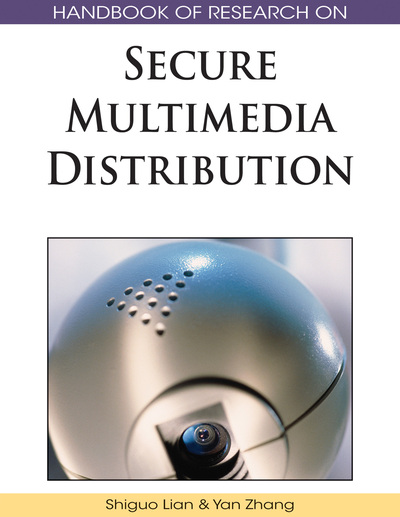# A Summary of Recent and Old Results on the Security of the Diffie-Hellman Key Exchange Protocol in Finite Groups

Ionut Florescu (Stevens Institute of Technology, USA)
DOI: 10.4018/978-1-60566-262-6.ch010
Available
\$29.50
No Current Special Offers

## Abstract

Regarding fundamental protocols in cryptography, the Diffie-Hellman (Diffie and Hellman, 1976) public key exchange protocol is one of the oldest and most widely used in today’s applications. Consequently, many specific cryptographic implementations depend on its security. Typically, an underlying (finite dimensional) group is selected to provide candidates for the key. The study of the security of the exchange as depending on the structure of the underlying group is even today poorly understood, with the most common approaches relying on the security of the Discrete Logarithm problem or on the size of the group. Recent developments bring to attention that the relationship is not necessarily valid and that more research is needed that will relate the underlying structure of the group and the security of the Diffie- Hellman exchange. In this chapter, we describe the problem in detail, we present the relationship with the previously studied Discrete Logarithm and Computational Diffie-Hellman problems, we expose the various concepts of security, and we introduce a new statistical concept specifically designed to serve the assessment of the security of the exchange.
Chapter Preview
Top

## Introduction

A key exchange protocol, is any algorithm through which two parties A and B agree on a common key. Once the key is established, any further information shared between the parties is encoded, transmitted and decoded using the key. The protocol is secure if any third party C finds it extremely hard (impossible in practice) to identify the key.

In a public key exchange protocol the two parties agree on a common key pooled from a set S while communicating over an insecure channel. The difference is that all the information exchanged over the insecure channel as well as the set of possible keys S is known by the perpetrator C. If C cannot tell apartfrom any other value in the set S, given the information observed guarantees that it is computationally unfeasible to gain “any” partial information on the key.

The Diffie-Hellman key exchange protocol (Diffie and Hellman, 1976) is a primary example of a public key exchange protocol. In its most basic form, the protocol chooses a finite cyclic groupof order N, with generator g, wheredenotes the group operation. In what follows we chose the multiplicative operation to denote the operation in the group, and thus the group G is generated by the powers of g (i.e.,), symbolically. Note that G, g and N are public information.

## Key Terms in this Chapter

Statistically Indistinguishable Random Variables: Are two or more random variable whose distribution is identical almost everywhere (with the possible exception of a set of probability measure zero).

Prime Group: A group that contains no subgroups except for the trivial subgroup. A prime subgroup is a subgroup of a group that contains no further subgroups except for the trivial subgroup. An example is included in.

Cryptographic Key: A piece of information that controls the operation of a cryptographic algorithm.

Generator of a Cyclic Group: An element g such that all the elements of the group are generated by successive applications of the group operation to g itself. Not all the elements in a group are generators.

p-Value of a Test: the probability of obtaining as extreme or more extreme values as the result of the experiment assuming that the null hypothesis is true. Numbers close to 0 are evidence against the null hypothesis (it is unlikely to see such numbers if the null hypothesis would be true).

Encryption Key: A piece of information used to specify the particular transformation of plaintext into ciphertext, or vice versa during the encryption/decryption process.

Subgroup of a Group: A set of elements from the initial group which together form a smaller goup structure included in the original group (i.e, the operation stays in the subgroup, the identity and the inverse elements are in the subgroup) . An example is the trivial subgroup .

## Complete Chapter List

Search this Book:
Reset